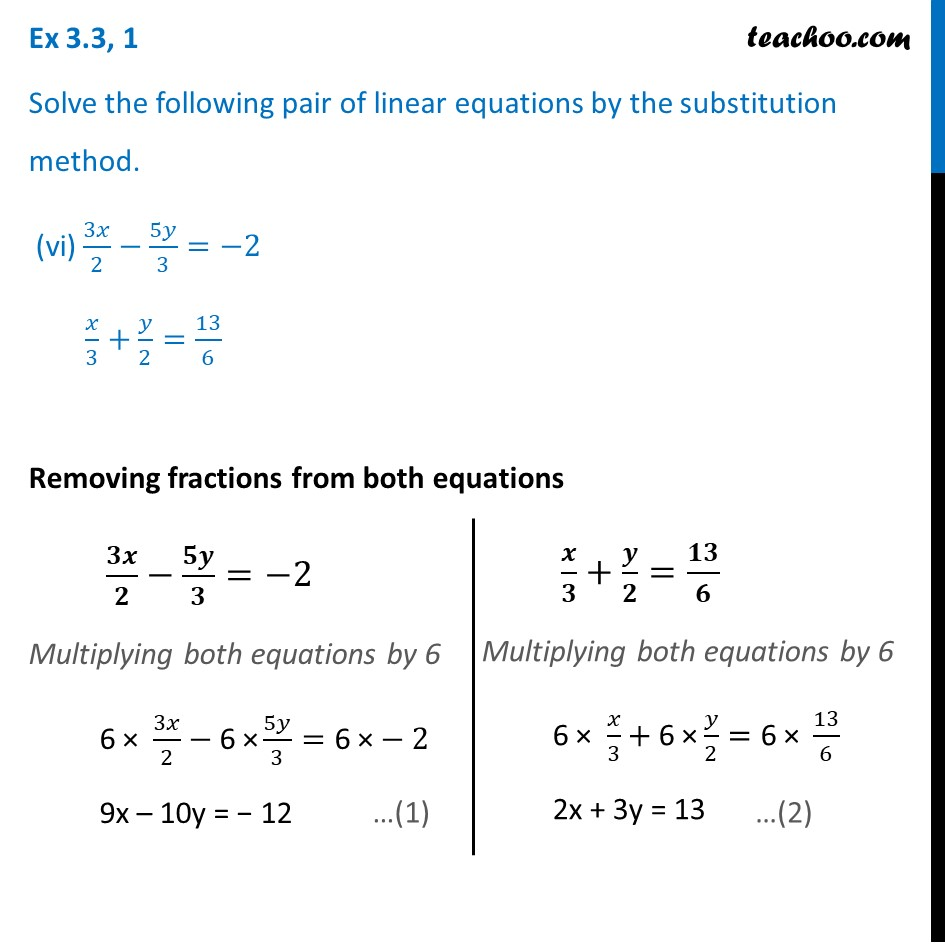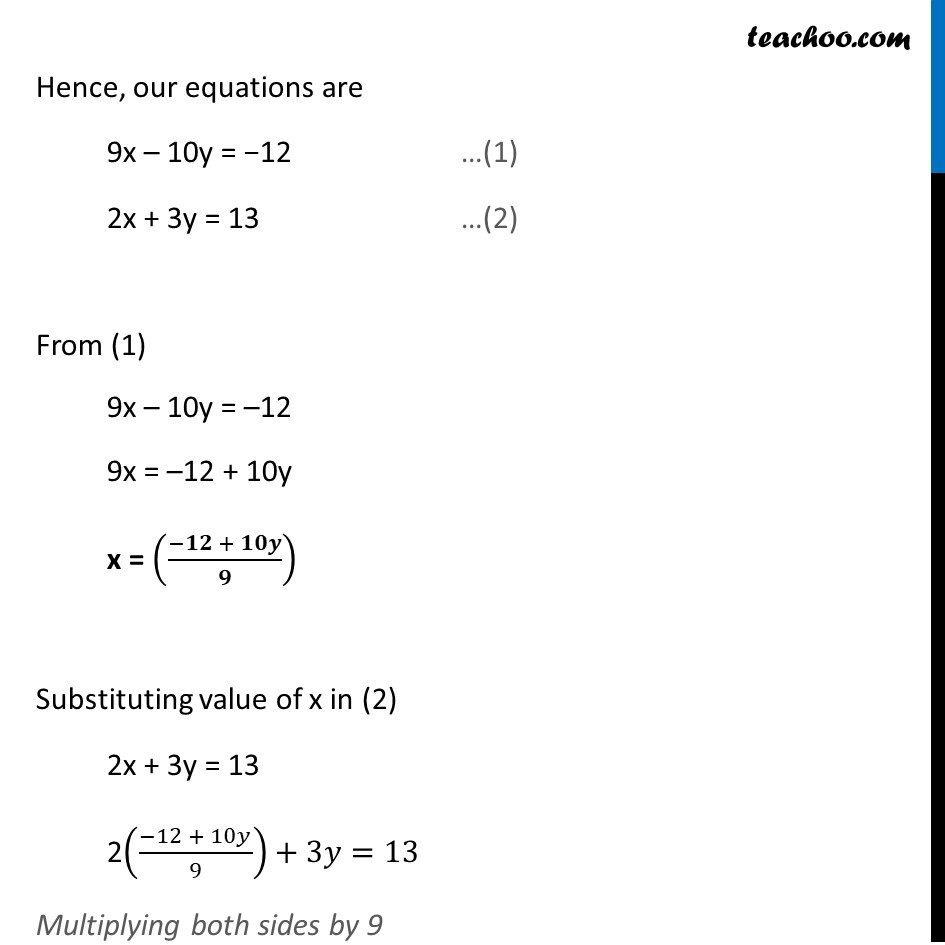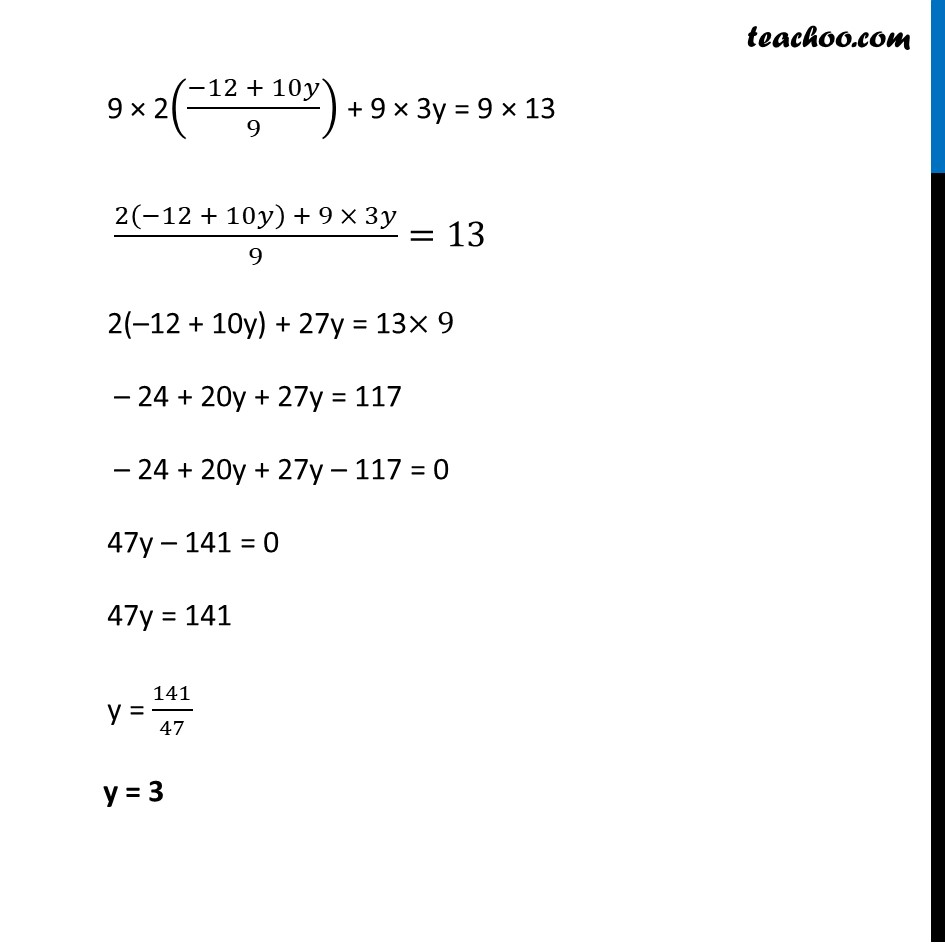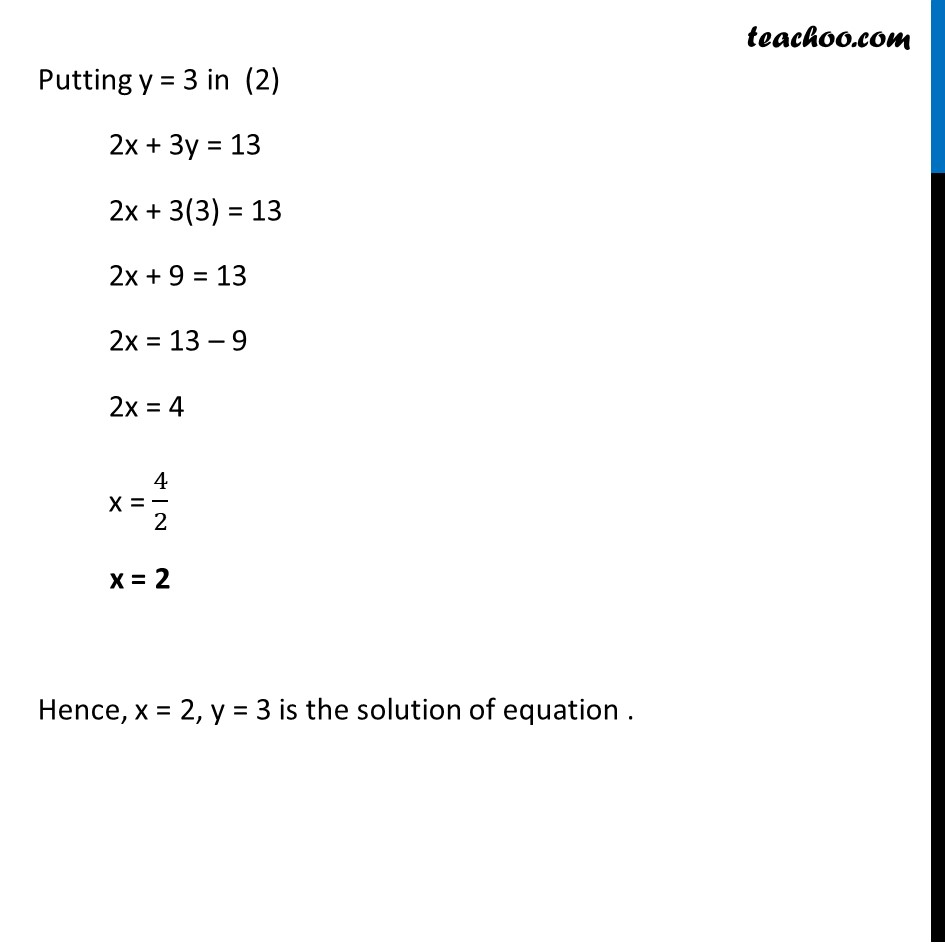Ex 3.3

Chapter 3 Class 10 Pair of Linear Equations in Two Variables
Serial order wiseGet live Maths 1-on-1 Classs - Class 6 to 12

### Transcript

Ex 3.3, 1 Solve the following pair of linear equations by the substitution method. (vi) 3𝑥/2−5𝑦/3=−2 𝑥/3+𝑦/2=13/6 Removing fractions from both equations 𝟑𝒙/𝟐−𝟓𝒚/𝟑=−2 Multiplying both equations by 6 6 × 3𝑥/2−"6 ×" 5𝑦/3="6 ×"−2 9x – 10y = − 12 𝒙/𝟑+𝒚/𝟐=𝟏𝟑/𝟔 Multiplying both equations by 6 6 × 𝑥/3+"6 ×" 𝑦/2="6 × " 13/6 2x + 3y = 13 Hence, our equations are 9x – 10y = −12 …(1) 2x + 3y = 13 …(2) From (1) 9x – 10y = –12 9x = –12 + 10y x = ((−𝟏𝟐 + 𝟏𝟎𝒚)/𝟗) Substituting value of x in (2) 2x + 3y = 13 2((−12 + 10𝑦)/9)+3𝑦=13 Multiplying both sides by 9 9 × 2((−12 + 10𝑦)/9) + 9 × 3y = 9 × 13 (2(−12 + 10𝑦) + 9 × 3𝑦)/9=13 2(–12 + 10y) + 27y = 13×9 – 24 + 20y + 27y = 117 – 24 + 20y + 27y – 117 = 0 47y – 141 = 0 47y = 141 y = 141/47 y = 3 Putting y = 3 in (2) 2x + 3y = 13 2x + 3(3) = 13 2x + 9 = 13 2x = 13 – 9 2x = 4 x = 4/2 x = 2 Hence, x = 2, y = 3 is the solution of equation .